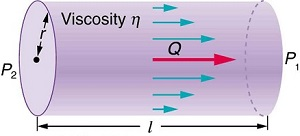Poiseuille’s law for resistanceResistance to laminar flow of an incompressible fluid in a tube. This formula is provided solely for hypothetical demonstration only.Contrast Media Viscosity (cP) @ 37°C Isovue 370 9.4 Omnipaque 350 10.4 Optiray 350 9.0 Vispaque 320 11.8

This is what you may feel with your hand using syring injection.

 Spanish SP Japanese JP

SoftNAV, 4F
Resistance = 8 x Viscosity x Length ÷ 3.14 x Radius4
= 8 x   x   ÷ 3.14 x
gram(s)/centimeter/second
1 centipoise = 0.01 gram/centimeter/second
Competitor, 4F
Resistance = 8 x Viscosity x Length ÷ 3.14 x Radius4
= 8 x   x   ÷ 3.14 x
gram(s)/centimeter/second
1 centipoise = 0.01 gram/centimeter/second
SoftNAV, 5F
Resistance = 8 x Viscosity x Length ÷ 3.14 x Radius4
= 8 x   x   ÷ 3.14 x
gram(s)/centimeter/second
1 centipoise = 0.01 gram/centimeter/second
Competitor, 5F
Resistance = 8 x Viscosity x Length ÷ 3.14 x Radius4
= 8 x   x   ÷ 3.14 x
gram(s)/centimeter/second
1 centipoise = 0.01 gram/centimeter/second